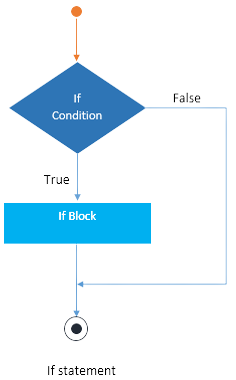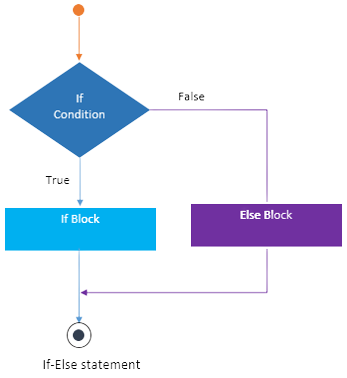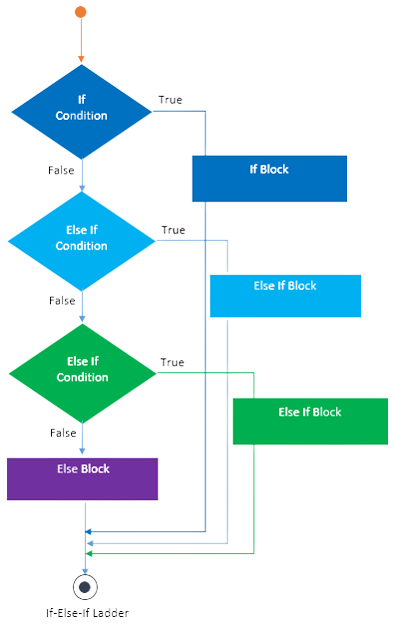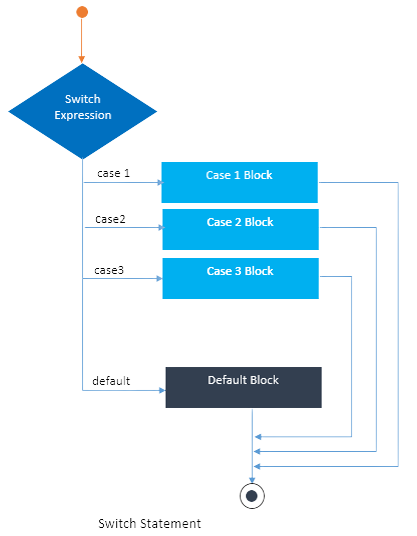# Conditional Statements in Java: If, If-Else and Switch Statement

22 Sep 2023
Beginner
1.8K Views

## Conditional Statements in Java: An Overview

Conditional statements in Java are one of the significant parts of "Control Structure" in Java. Conditional statements are based on certain conditions and generate decisions accordingly. These statements are a bunch of codes that can be executed by "decision statements" which are crucial. These conditions have some specific "boolean expressions," representing expressions that evaluate to either true or false, aiding beginners in understanding Java programming concepts. To make it easier these Java for beginners tutorials to grasp the concepts including conditional statements in Java, java conditional operator, Java Conditional Statements, Conditional statements in Java examples, and Java if statement with Examples.

## What are Conditional Statements in Java?

Conditional statements are one of the significant parts of "Control Structure" in Java. Conditional statements are based on certain conditions and generate decisions accordingly. These statements are a bunch of codes that can be executed by "decisions statements". These conditions have some specific "boolean expressions". The boolean expression of these conditional statements generates "Boolean Value" which could be either true or false.

## Types of Conditional Statements in Java

There are 4 types of conditional statements in Java discussed in this Beginner’s Guide to Java. They are if statements in Java, if else statements in Java, ladder statements or If Else If statements, and Switch statements. The types of conditional statements in Java will be discussed further.

### If..statement in Java

• “If” is a statement execution that depends on certain conditions.
• These conditions only follow the "if" keyword.
• The "If" statement depends on the expression of a certain boolean and generates a Boolean Value.
• If the value is "True" then the execution will be done in the current block.
• But if the Value is "false", it will switch to the next statement.#### Syntax

``````If (condition)
{
//Statements to be executed
}``````

#### Java if else example

``````class if_condition
{
public static void main(String[] args)
{
double a = 0.8;
if (a>0)
{
System.out.println(a + " is a Positive Number!");
}
}
}``````

This Java code assigns the value 0.8 to the variable "a," and a "if" condition determines whether "a" is greater than 0. If accurate, it confirms that 0.8 is a positive number by printing "0.8 is a Positive Number!" to the console.

#### Output

``0.8 is a Positive Number!``

### If..else statement in Java

• The if else statement Java is a particular control structure that depends on selecting the conditions of the chosen set of statements.
• The if else program in Java depends on two types of conditions, which are "If" and "Else".
• If the expression generates the "true" value then it will execute the block "If" in the if-else program in the if else program in Java.
• But if the value is "False", it will execute the "Else" block which depends on the if-else condition.#### Syntax

``````if (condition)
{
//Statements to be executed if condition satisfies
}
else
{
//Statements to be executed if the condition is not satisfied
}
``````

#### If-else example in Java

``````class if_else_condition
{
public static void main(String[] args)
{
double a = -0.8;
if (a>0)
{
System.out.println(a + " is a Positive Number!");
}
else
{
System.out.println(a + " is a Negative Number!");
}
}
}``````

This Java code gives the value -0.8 to the variable "a". It determines whether "a" is greater than 0 by using a "if-else" condition. If true, the console displays "0.8 is a Positive Number!"; if false, it displays "0.8 is a Negative Number!" to indicate that -0.8 is a negative number.

#### Output

``-0.8 is a Negative Number!``

### If..else...If statement in Java

• This control structure statement depends on a series of tests in order to evaluate the solution
• It is generated by the multiple uses of the "If Else" statement.
• If the one condition meets the result then the first ladder will be executed.
• But if the condition does not meet the results then the default "Else" statement will be executed.#### Syntax

``````if (condition)
{
//Statements set 1
}
else if (condition 2)
{
//Statements set 2
}
. . .
else
{
//Statements to be executed if no condition is satisfied.
}
``````

#### Example

``````class nested_if_else_condition
{
public static void main(String[] args)
{
double total_marks = 382;
double perc = (total_marks/500)*100;
if (perc >= 80)
{
}
else if ((perc >=70) && (perc <80))
{
}
else if ((perc >=60) && (perc <70))
{
}
else
{
}
System.out.println("The percentage of the student is: " +perc);

}
}``````

With the help of this Java program, you can figure out a student's percentage, give them a grade based on predetermined ranges, and print out both the % and the grade. In this instance, the "nested_if_else_condition" class, which assesses student performance, would output the percentage and grade based on the 382 total marks.

#### Output

``````The percentage of the student is: 76.4
The grade of the student is: A+``````

### Switch Statement in Java

• This statement has multiple phases of execution. Not only that In Java, access modifiers play a crucial role in controlling the visibility of variables, methods, and classes.
• Switch statements generally evaluate the result which is assisted by some "primitive type" of data or "class type" of data.
• This statement has a test series of conditions that can do one or more cases at a time by switching expressions.
• If the case meets the result then the statement will be executed.
• But if it does not meet the result then the "default" cases will be executed.#### Syntax

``````switch (Expression)
{
case value 1: Statement 1;

case value 2: Statement 2;

case value 3: Statement 3;
.
.
.
case value n: Statement n;

default: default statement;
}``````

#### Example

``````class Switch_Case {
public static void main(String[] args)
{
char a = 'C';
switch (a)
{
case 'A':
System.out.println("Letter A");
break;
case 'C':
System.out.println("Letter C");
break;
default:
System.out.println("Default case: NO Letter Matched!");
}
}
}``````

The switch-case statement is used in this Java code example. Because it matches the 'C' case, it examines the value of the character variable "a" and prints "Letter C," but the default case is not used.

#### Output

``Letter C``

## If..else vs. Switch statements

Parameter If...Else Switch
Definition The execution of both of the "If" blocks and "else", depends on the condition stored in the if statement. The code blocks in the switch statement depend on the execution of multiple cases.
Usage This statement is used for pointer, character, integer, a boolean type, and floating point type. Switch statements are used for integer and character expressions
Testing It tastes equality and logical expression. It tests only equality
Expressions It generates multiple statements for multiple decisions. It generates a single expression for multiple decisions.
Editing It is difficult to edit because of its nested type of program. It is very easy to edit
Value It is dependent on constraint. It is dependent on users.

## FAQs

### 1. What are the 4 conditional statements in Java?

In Java, there are four types of conditional statements: "if," "if-else," "else-if," and "switch."

### 2. What are conditional statements in Java?

Java conditional statements are control structures that let you run several code blocks in accordance with predefined conditions.

### 3. How to write 3 conditions in an if statement in Java?

Multiple "else-if" clauses can be used in Java to write three conditions in a "if" statement.

### 4. What is the syntax of a conditional statement?

if (condition) {

// Code block to execute if the condition is true

}

### 5. What are the advantages of conditional statements?

Making code decisions, managing various scenarios, and permitting dynamic behaviour, which boosts programme flexibility and functionality, are all benefits of conditional expressions in Java.

##### Summary

This article gives a vast idea about "Java for beginners" on the definition of a conditional statement and its types. Features of every conditional operator in Java with examples and syntax are explained. This article also includes the different types of conditional statements in Java and the difference between the If-else condition and the switch condition.

Similar Articles